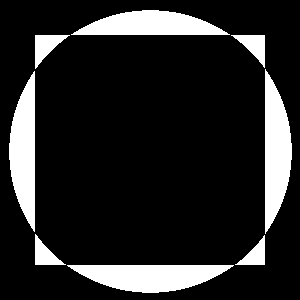# danangell.com/blog

A common riddle-like question for programmers asks them to swap the values of two integers without a temporary intermediate value. There are two common solutions that I’m aware of, addition swap and XOR swap. Here’s what each looks like in C:

void addSwap (unsigned int *a, unsigned int *b) {
if (a != b) {
*a = *a + *b;
*b = *a - *b;
*a = *a - *b;
}
}

void xorSwap (int *a, int *b) {
if (a != b) {
*a ^= *b;
*b ^= *a;
*a ^= *b;
}
}


Credit: https://en.wikipedia.org/wiki/XOR_swap_algorithm

The more interesting of the two is XOR swap. It only uses a single operation, rather than addition and subtraction in addition swap. Wikipedia tries to explain this with bit vectors but I think 1 bit black and white images work better. In the images below, black corresponds to 0 and white corresponds to 1.

A ^ B = A':

Here A becomes white anywhere A or B had a unique presence (only A or only B was black in that pixel). Everywhere else is black.

A B A'A' ^ B = B':

Now that A is a combination of what was unique in each of the original images, we can apply the same XOR operation to remove what was unique about B, leaving only what was unique about A. So at this point B has swapped and become the circle.

A' B B'A' ^ B' = A'':

We can perform the same operation as in the previous step to get back the original value of B. Since B holds the circle and we want the square, we remove what was unique about the circle, leaving just the square.

A' B' A''Here’s the code used to generate the images above:

import sys
from PIL import Image

a = Image.open("A.png")
b = Image.open("B.png")

if not a.size == b.size:
print("Images must have the same dimensions")
sys.exit(1)

if not a.mode == b.mode:
print("Images must be the same mode")
sys.exit(1)

result = bytes([
a.getpixel((x, y)) ^ b.getpixel((x, y))
for y in range(a.size)
for x in range(a.size)
])

Image.frombytes("P", a.size, result).save("OUT.png")